# Meaning of partial derivative depends on entire coordinate system

## Statement

### Mathematical statement

Consider a function of more than one variable. Then, the partial derivative with respect to one variable depends not only on the choice of that particular variable, but on the choice of the other variables that are being kept constant for the purpose of computing the partial derivative. In particular, if a coordinate transformation is performed that changes what those other variables are, that could affect the value of the partial derivative.

### Real-world statement

This has a very real-world corollary. In economics and social science, we often talk of the partial derivative with respect to one variable as measuring what happens ceteris paribus on the other variables. However, the notion of ceteris paribus on other variables depends on what the other variables are. If we redefine the coordinate system to change that meaning, the partial derivative can change.

## Mathematical examples

### Linear function of two variables and linear change of variables

Consider the function: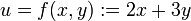$u = f(x,y) := 2x + 3y$

In this case, we have:$\frac{\partial u}{\partial x} = f_x(x,y) = 2$

Now, suppose we consider$f$ in terms of$x$ and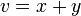$v = x + y$. Then, we have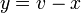$y = v - x$. Rewriting$u$ in terms of$x$ and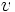$v$, we get: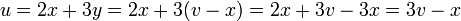$u = 2x + 3y = 2x + 3(v - x) = 2x + 3v - 3x = 3v - x$

In other words, we can define$u$ as a function of two variables$x$ and$v$. If we use the letter$g$ to denote this new function, we get: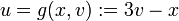$u = g(x,v) := 3v - x$

In this case, we have: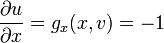$\frac{\partial u}{\partial x} = g_x(x,v) = -1$

Note that the two partial derivatives with respect to$x$ are not equal. The reason for this is that in the first case, we are taking the partial derivative with respect to$x$ keeping$y$ constant, whereas in the second case, we are taking the partial derivative with respect to$x$ keeping$v = x + y$ constant. In this case, when we increase$x$ slightly, the value of$y$ decreases to keep the total constant.

Here's the geometric interpretation:

• In the first case, where we are computing$f_x(x,y)$, we are geometrically computing the directional derivative along the positive$x$-direction, i.e., along a line with$y$-coordinate.
• In the second case, where we are computing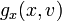$g_x(x,v)$, we are geometrically computing (up to scalar multiples) the directional derivative along lines with$x + y$ constant. These lines are downward sloping with a slope of$-1$.
ASIDE: VARIABLE AND FUNCTION NOTATION: If we are using the function notation, we need to give different names to the function depending on the coordinate system for the inputs. In the above example, we used the letter$f$ to describe the function in the original coordinate system and$g$ to describe the function in the new coordinate system. However, the notation for the variable that we use to describe the output of the function remains the same. In the above example, it is$u$ in both coordinate systems.

This example is deliberately kept simple because we chose linear functions and linear transformations, so the partial derivatives were all constants. Subsequent examples consider a more complicated situation.

### Quadratic function of two variables and linear transformation

Consider the function: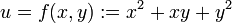$u = f(x,y) := x^2 + xy + y^2$

The partial derivative with respect to$x$ is:$\frac{\partial u}{\partial x} = f_x(x,y) = 2x + y$

Consider$v = x + y$. We then have$y = v - x$. Let's attempt to write$u$ in terms of$x$ and$v$:$u = x^2 + x(v - x) + (v - x)^2 = x^2 + xv - x^2 + v^2 - 2xv + x^2 = x^2 - xv + v^2$

If we denote this new function by$g$, we obtain:$u = g(x,v) := x^2 - xv + v^2$

The partial derivative with respect to$x$ is: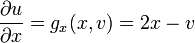$\frac{\partial u}{\partial x} = g_x(x,v) = 2x - v$

Note that as of now, the two partial derivatives with respect to$x$ are not directly comparable because one is an expression in terms of$x,y$ and the other is an expression in terms of$x,v$. However, we can convert the expression in terms of$x,v$ back to an expression in terms of$x,y$ by plugging back$v = x + y$. We get:$\frac{\partial u}{\partial x} = g_x(x,v) = 2x - (x + y) = x - y$

We now see that the two partial derivative expressions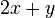$2x + y$ and$x - y$ are distinct, and they coincide only for points on the line$x + 2y = 0$, which can be written as$y = \frac{-1}{2}x$.

## Keeping track of the issue

The actual method for figuring out partial derivatives in the new coordinate system, using partial derivatives in the old coordinate system, uses a matrix called the Jacobian. This matrix basically uses the chain rule for partial differentiation to move between the old and new coordinate systems. The fact that some of the variables are not being changed is captured by some of the entries in the Jacobian being 1 and some of the entries being 0.

## Real-world examples

### Transformation between averages and products

Suppose a country's military spending is determined by just two factors: its per capita GDP and its population. We want to study the relationship between per capita GDP and military spending.

There are two sensible ways (among many) of trying to do this:

• Study the relationship between per capita GDP and military spending holding population constant. In other words, take the partial derivative with respect to per capita GDP holding population constant. In this case, we are thinking of military spending as a function of the two variables: per capita GDP, and population.
• Study the relationship between per capita GDP and military spending holding total GDP constant. Prima facie, this is similar to the previous one, because total GDP is just a product of per capita GDP and population. However, what we have done effectively is consider a partial derivative of the function in a new coordinate system, where the two variables of interest are: per capita GDP, and (per capita GDP) times (population).

The point is that the partial derivatives will have different expressions depending on what we hold constant. They will be related (in fact, we can relate them through an application of the chain rule for partial differentiation and product rule for differentiation). However, it's possible that one of them is positive and the other negative, or that one of them is zero and the other nonzero. The upshot is that to understand what a partial derivative means, we need to know not just the variable with respect to which differentiation occurs but also the coordinate system, i.e., the meaning of the partial derivative depends on the choice of other variables being kept constant.